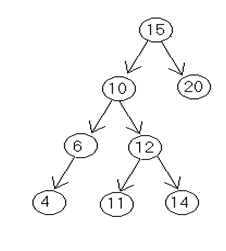## Algorithm to delete the specific node from binary searchtree, Data Structure & Algorithms

Assignment Help:

Q. Write down an algorithm to delete the specific node from binary search tree. Trace the algorithm to delete a node (10) from the following given tree.Ans.

Algorithm for Delete ting the specific Node From the Binary Search Tree

To delete the specific node following possibilities may arise

1)      Node id a terminal node

2)      Node have only one child

3)      Node having 2 children.

DEL(INFO, LEFT, RIGT, ROOT, AVAIL, ITEM)

A binary search tree T is in the memory, and an ITEM of information is given as follows.
This algorithm deletes the specific ITEM from the tree.

1. [to Find the locations of ITEM and its parent] Call FIND(INFO, RIGHT, ROOT, ITEM, LOC, PAR).

2. [ITEM in tree?]

if LOC=NULL, then write : ITEM not in tree, and Exit.

3. [Delete node containing ITEM.]

if RIGHT[LOC] != NULL and LEFT[LOC] !=NULL then:

Call CASEB(INFO,LEFT,RIGHT,ROOT,LOC,PAR). Else:

Call CASEA (INFO,LEFT,RIGHT,ROOT,LOC,PAR).

[End of if structure.]

4. [Return deleted node to AVAIL list.] Set LEFT[LOC]:=AVAIL and AVAIL:=LOC.

5. Exit.

CASEB(INFO,LEFT,RIGHT,ROOT,LOC,PAR)

This procedure will delete the node N at LOC location, where N has two children. The pointer PAR gives us the location of the parent of N, or else PAR=NULL indicates that N is a root node. The pointer SUC gives us the location of the inorder successor of N, and PARSUC gives us the location of the parent of the inorder successor.

1. [Find SUC and PARSUC.]

(a) Set PTR: = RIGHT[LOC] and SAVE:=LOC. (b) Repeat while LEFT[PTR] ≠  NULL:

Set SAVE:=PTR and PTR:=LEFT[PTR]. [End of loop.]

(c) Set SUC : = PTR and PARSUC:=SAVE.

2. [Delete inorder successor]

Call CASEA (INFO, LEFT, RIGHT, ROOT, SUC, PARSUC).

3. [Replace node N by its inorder successor.] (a) If PAR≠NULL, then:

If LOC = LEFT[PAR], then: Set LEFT[PAR]:=SUC.

Else:

Set RIGHT[PAR]: = SUC. [End of If structure.]

Else:

Set ROOT: = SUC. [End of If structure.]

(b) Set LEFT[SUC]:= LEFT [LOC] and

RIGHT[SUC]:=RIGHT[LOC]

4. Return.

CASEA(INFO, LEFT, RIGHT, ROOT, LOC, PAR)

This procedure deletes the node N at LOC location, where N does not contain two children. The pointer PAR gives us the location of the parent of N, or else PAR=NULL indicates that N is a root node. The pointer CHILD gives us the location of the only child of the N, or else CHILD = NULL indicates N has no children.

1. [Initializes CHILD.]

If LEFT[LOC] = NULL and RIGHT[LOC] = NULL, then: Set CHILD:=NULL.

Else if LEFT[LOC]≠NULL, then:

Set CHILD: = LEFT[LOC].

Else

Set CHILD:=RIGHT[LOC] [End of If structue.]

2. If PAR ≠  NULL, then:

If LOC = LEFT [PAR], then:

Set LEFT[PAR]:=CHILD.

Else:

Set RIGHT[PAR]:CHILD = CHILD [End of If structure.]

Else:

Set ROOT : = CHILD.

[End of If structure.]

3. Return.

Inorder traversal of the tree is

4 6 10 11 12 14 15 20

To delete 10

PAR = Parent of 10 ie 15

SUC = inorder succ of 10 ie. 11

PARSUC = Parent of inorder succ ie 12

PTR = RIGHT [LOC]

CHILD = NULL

LEFT [PARSUC] = CHILD= NULL LEFT [PAR]= ADDRESS OF 11

LEFT [SUC] = LEFT [LOC] = ADDRESS OF 6

RIGHT [SUC] = RIGHT[LOC] = ADDRESS OF 12

#### Pseudo code, since the gregorian calendar was introduced in 1752,a leap yea...

since the gregorian calendar was introduced in 1752,a leap year occurs every 4 years.you are to write a pseudo code to find out whether a year is a leap year.your progrm should dis

#### Algorithm for determining who won rock paper scissors game, Suppose you are...

Suppose you are given the results of 5 games of rock-paper-scissors. The results are given to you on separate pieces of paper; each piece says either 'A' if the first person won, o

#### Algorithm for dfs, Step 1: Choose a vertex in the graph and make it the sou...

Step 1: Choose a vertex in the graph and make it the source vertex & mark it visited. Step 2: Determine a vertex which is adjacent to the source vertex and begun a new search if

#### Which is the most suitable data type, Problem 1. You are asked to store...

Problem 1. You are asked to store Names of all 100 students of class A in your Learning Centre. Which data type will you use? What is its syntax? Explaining the data typ

#### Infix notation to postfix notation, Which data structure is required to cha...

Which data structure is required to change infix notation to postfix notation?    Stack function is used to change infix notation to postfix notatio n

#### Algorithm, algorithm to search a node in linked list

algorithm to search a node in linked list

#### Threads in main method, Create main method or a test class that creates 2 E...

Create main method or a test class that creates 2 Element objects that are neighbours of each other, the first element temperature set at 100, the 2nd at 0 and use an appropriate h

#### Binary search tree, bst for 40,60,25,50,30,70,35,10,55,65,12

bst for 40,60,25,50,30,70,35,10,55,65,12

#### Memory allocation strategies, Q. Explain the various memory allocation stra...

Q. Explain the various memory allocation strategies.                                                            Ans. M e m ory Allocation Strategies are given as follow

#### Postfix expression, : Write an algorithm to evaluate a postfix expression. ...

: Write an algorithm to evaluate a postfix expression. Execute your algorithm using the following postfix expression as your input: a b + c d +*f ­ .